# Unit Digit – Download Mathematics Study Notes Free PDF For REET/UTET Exam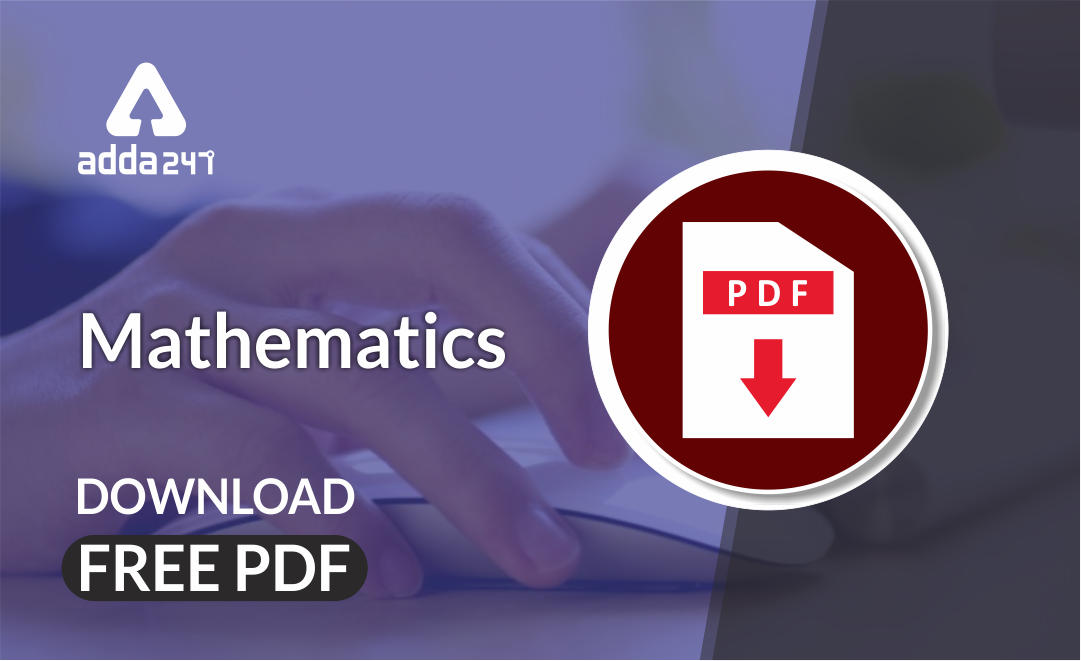Mathematics is an equally important section for  REETMPTET & DSSSB Exams and has even more abundant importance in some other exams conducted by central or state govt. Generally, there are questions asked related to basic concepts and formulas of the Mensuration. To let you make the most of Mathematics section, we are providing important facts related to the Unit Digit. At least 2-3 questions are asked from this topic in most of the teaching exams. We wish you all the best of luck to come over the fear of the Mathematics section.

Mathematics Study Notes For All Teaching Exams

## Unit Digit

In the exams like CTET or State TET, the Mathematics section is the most scoring section among all. Students can score full marks in the Mathematics section if they manage their time properly in the exam. Questions may seem time-consuming but shortcut methods can save our crucial time. Students who are unaware of the shortcut methods can’t beat time.

Question: Find the unit place digit in (657)85 – (158)37

## TIPS AND TRICKS TO FIND UNIT DIGIT

Numbers are classified into three categories to find unit digit.

1. Digits 0,1,5,6
2. Digits 4,9
3. Digits 2,3,7,8

## Digits 0,1,5,6

When we have these numbers (0,1,5,6) in the unit place, we get the same digit itself at the unit place when raised to any power, i.e. 0n=0, 1n=1, 5n=5, 6n=6. Let us apply this concept to the following questions.

Example: Find the Unit place digit of the following numbers:

1. 360244

1. 2974281307

1. 4575400000666

1. 5687686265749375

How to Overcome Exam Fever, Especially When You Fear Maths

## Digits 4 & 9

Both these numbers have a cyclicity of only two different digits as their unit’s digit.

In the case of 4 & 9

• If the Power of 4 is Even, the result will be 6
• If the Power of 4 is Odd, the result will be 4
• If the Power of 9 is Even, the result will be 1
• If the Power of 9 is Odd, the result will be 9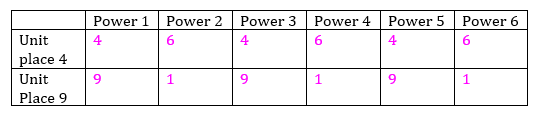Example: Find the Unit place digit of the following numbers:

1. 456847426734258

1. 3456445767843

1. 548574657895768454

1. 4576348567895627369765787

Get free Study material for REET Exam

## Digits 2,3,7,8

For Digit 2

When we have number 2 in the unit place then follow the given steps to find the unit digit.

Step 1- Divide the last two digits of the power of a given number with 4

Step 2- You get the remainder n

Step 3- Since you have got n as a remainder, put it as the power of 2, i.e (2)n

Step 4- Have a look at the table below and mark your answer.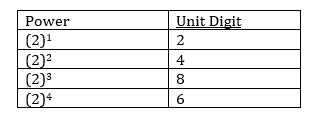Example: Find the Unit place digit of the following numbers:

1. 4657233

Here, the unit place is 2 and power is 33. To solve follow the given steps

Step 1- Divide 33 by 4.

Step 2- You get remainder 1.

Step 3- Since you have got remainder 1, put it as a power of 2, i.e 21

Step 4- Have a look at the table above, 21=2.

1. 7657845678235

Here, the unit place is 2 and power is 33. To solve follow the given steps

Step 1- Divide 35 by 4.

Step 2- You get remainder 3.

Step 3- Since you have got remainder 3, put it as a power of 2, i.e 23

Step 4- Have a look at the table above, 23=8.

Practice REET Previous Year Maths Quiz For REET Exam Here

### For the digits 3,7,8

Repeat the steps

When we have the numbers 3,7,8 in the unit place then follow the given steps to find the unit digit.

Step 1- Divide the last two digits of the power of a given number with 4

Step 2- You get the remainder n

Step 3- Since you have got n as a remainder, put it as the power of 3,7,8, i.e 3n, 7n, 8n

Step 4- Have a look at the table below and mark your answer.

For Digit 3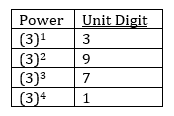Example: Find the Unit place digit of the following numbers:

1. 4657333

Here, the unit place is 3 and power is 33. To solve follow the given steps

Step 1- Divide 33 by 4.

Step 2- You get remainder 1.

Step 3- Since you have got remainder 1, put it as a power of 3, i.e 31

Step 4- Have a look at the table above, 31=3.

For Digit 7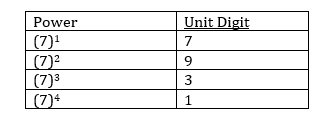Example: Find the Unit place digit of the following numbers:

1. 4657718

Here, the unit place is 7 and power is 18. To solve follow the given steps

Step 1- Divide 18 by 4.

Step 2- You get remainder 2.

Step 3- Since you have got remainder 2, put it as a power of 7, i.e 72

Step 4- Have a look at the table above, 72=9.

For Digit 8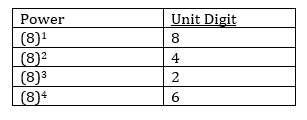Example: Find the Unit place digit of the following numbers:

1. 4657859

Here unit place is 8 and power is 59. To solve follow the given steps

Step 1- Divide 59 by 4.

Step 2- You get remainder 3.

Step 3- Since you have got remainder 3, put it as a power of 8, i.e 83

Step 4- Have a look at the table above, 83=2.

•अलंकार - परिभाष�...
•विशेषण- परिभाष�...
•लेखन कौशल की पर�...
•Complete CTET Child Development and Peda...
•New Education Policy(NEP) PDF - नई �...
•पठन कौशल- परिभा�...